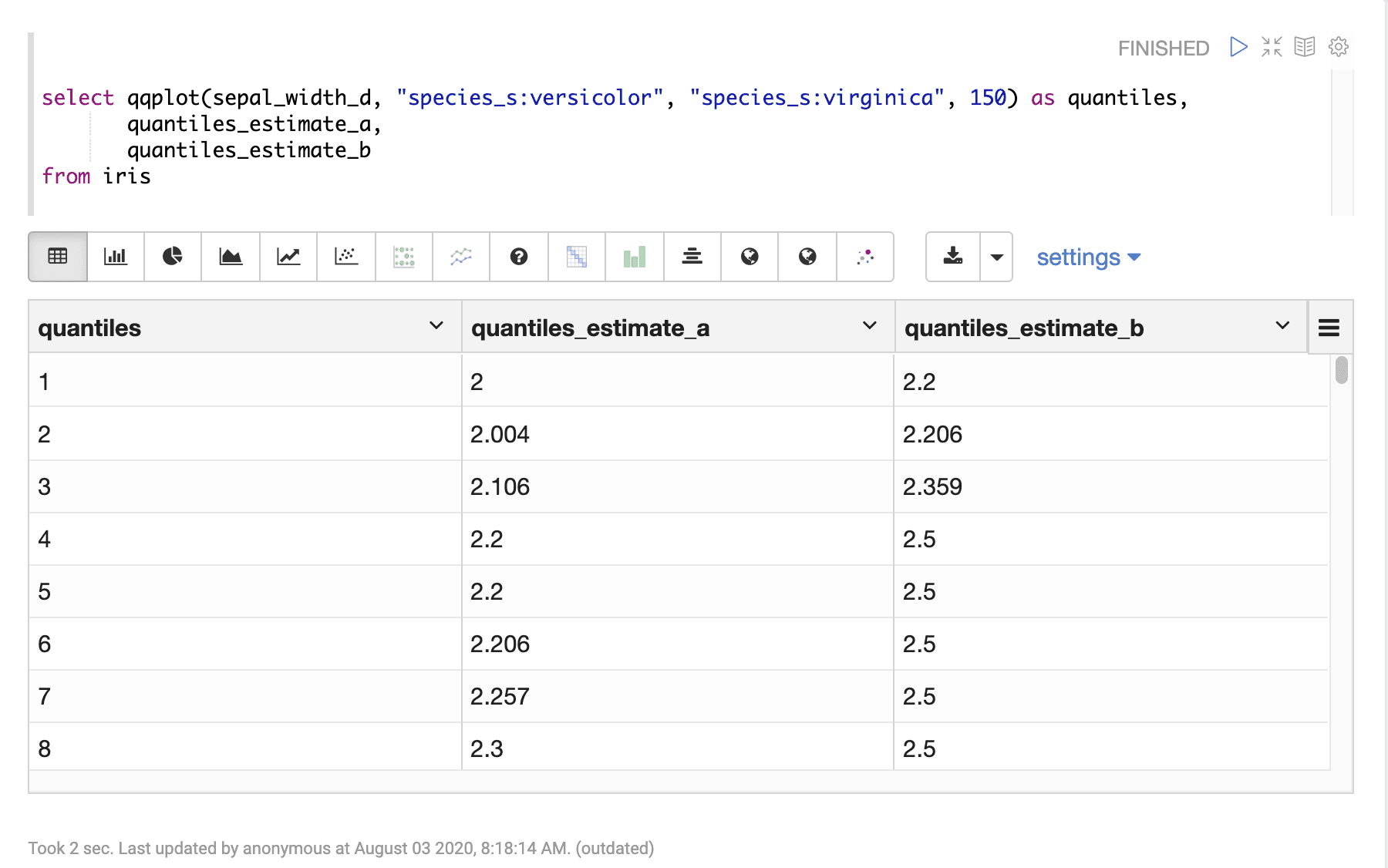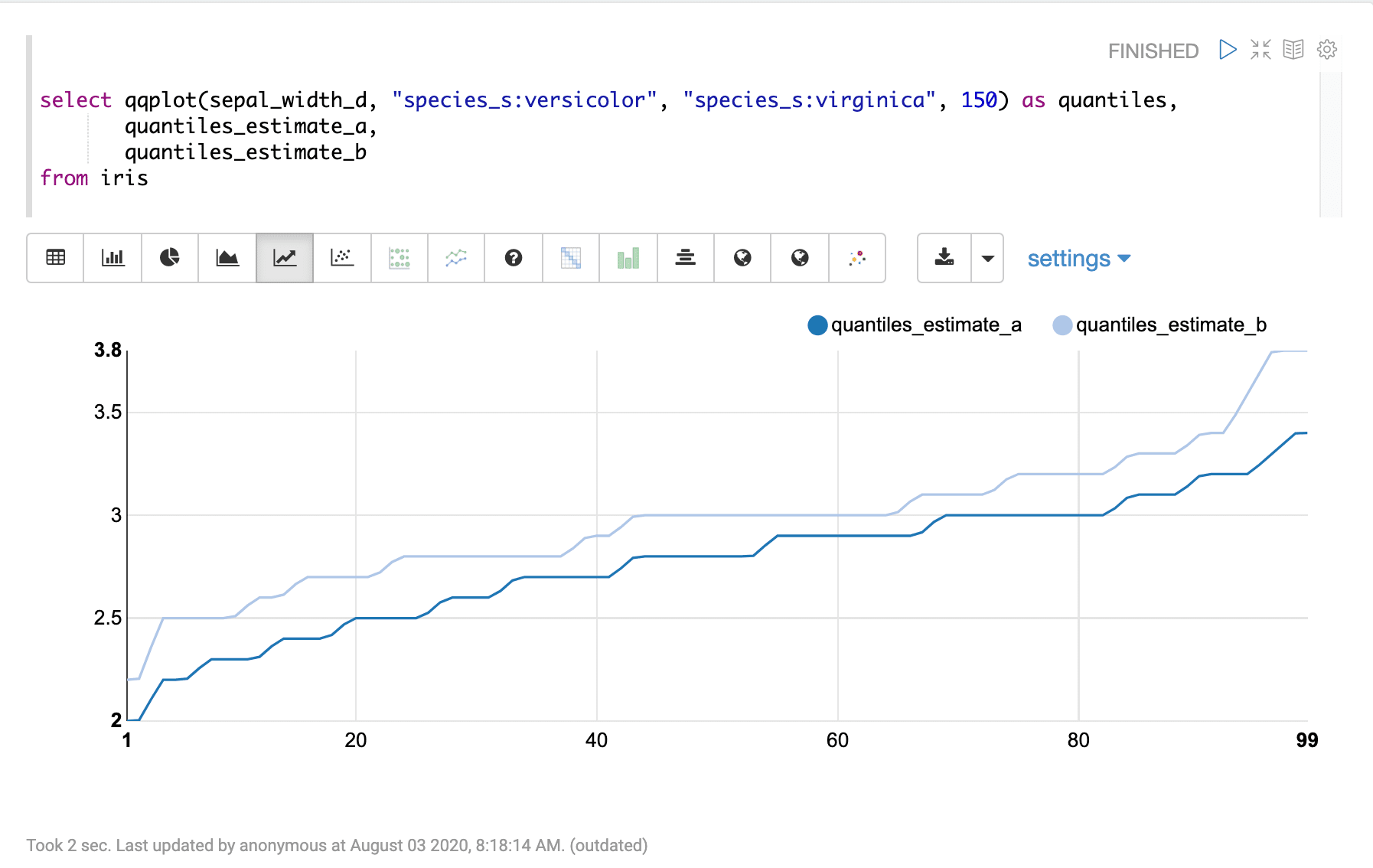# QQPlot

The `qqplot` function calculates percentiles (1–99) for two sample sets, based on queries, so they can be compared visually. The `qqplot` function takes four parameters:

1. The numeric field for which to calculate the percentiles

2. The Lucene/Solr query for the sample set A

3. The Lucene/Solr query for the sample set B

4. The sample size for both sample sets

## Sample syntax

``````select qqplot(sepal_width_d, "species_s:versicolor", "species_s:virginica", 150) as quantiles,
quantiles_estimate_a,
quantiles_estimate_b
from iris``````

## Result set

The result set for the `qqplot` function contains one row for each percentile 1–99. The `qqplot` function returns the percentile (1–99). The `quantiles_estimate_a` and `quantiles_estimate_b` fields contain the estimated percentile value, for sample sets A and B, at each percentile.

Sample result set in Apache Zeppelin## Visualization

The qqplot function can be visualized by plotting the percentile (1-99) on the x-axis and the quantiles_estimate_a and quantiles_estimate_b columns on the y-axis.

Sample visualization of percentiles in Apache Zeppelin